# Alternating Current Induction Generators## Overview

The alternating current (AC) induction machine is ubiquitous in modern life, by far the most widely used of all electric machines. The success of the induction machine can be attributed to its simple, rugged and efficient design. Although the induction design dominates motor applications, induction generators have only recently entered into widespread use.

Unlike synchronous machines, induction machines do not have circuitry to initiate or maintain excitation (magnetic flux) within the machine. This applies to both induction motors and generators. To maintain excitation, reactive power must be supplied by an overexcited synchronous machine or a shunt capacitor. Lacking internal excitation induction generators cannot perform black start duties to begin the power recovery process. Induction generators also cannot maintain AC system frequency or voltage. These limitations constrain their application as generators. 

The key advantage of an induction generator is the ability to tolerate a prime mover with varying angular velocities. Provided the power system can supply the reactive power requirements of the machine, induction generators can be used in power recovery applications, wind turbines and other irregular prime movers.

## Induction Machine Operation

The winding of the stator induces a rotating magnetic field within the motor in synchronism with the AC system frequency. When the induction machine is operated as a motor, the stator’s rotating magnetic flux induces a voltage and current in the rotor. The rotor is slotted and looks like a cage, hence the term squirrel cage rotor. When current is applied to the stator but the rotor is not spinning, the slip is unity,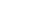. The magnetic field induced in the rotor starts to follow the magnetic field of the stator and the slip decreases.

When operating as a motor, the rotor’s magnetic field always lags behind that of the stator. If the rotor was spinning at the same speed as the magnetic field in the stator (synchronous speed,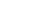) the induced voltage and current in the rotor would drop to zero and, the magnetically induced torque to turn the rotor would cease to exist. Ignoring resistive and mechanical losses, effectively no power is consumed with zero slip.

Even unloaded, an induction motor is always slipping relative to synchronous speed by several percent. This slip is necessary to maintain torque within the machine. As the load on the induction motor increases, the slip increases and thereby the strength of the magnetic torque on the rotor.Nameplate of a 100 HP, 480 VAC, 8 pole induction motor. The synchronous speed of this machine is 900 RPM but the rated speed under load is 880 RPM. The rotor slips with respect to the stator.

If a prime mover accelerates the rotor faster than synchronous speed, the slip becomes negative and the polarity of the induced voltage and current in the rotor reverses. With negative slip, the induction machine is begins supplying real power back to the power system. The machine is now acting as a generator. 

## Induction Generator Calculations

The equivalent circuit of an induction machine is shown below where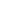is the stator resistance,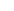is the stator reactance,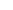is the rotor resistance, expressed in terms of the stator voltage and frequency,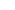is the rotor reactance, expressed in terms of the stator voltage and frequency,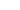is the magnetizing branch reactance andis the slip between the rotor and the stator.The equivalent circuit of an induction machine. This circuit is useful to calculate the performance of the machine under various operating conditions. r_1 is the stator resistance, x_1 is the stator reactance, r_2 is the rotor resistance, x_2 is the rotor reactance, x_m is the magnetizing branch reactance and s is the slip.

The following manufacturer-provided resistance and reactance values for the equivalent circuit of a 7 MW (9387 HP), 13.8 kV, 4-pole, 3 phase, 60 Hz squirrel cage induction machine will be used in subsequent calculations. Values are for a machine running at 110 ℃. Operating as a motor the machine draws 310 A with an efficiency of 96.6 % and a power factor of 0.94. Note thatwas not provided.: 0.11144 Ω: j3.0933 Ω: 0.13214 Ω: j1.7263 Ω: j189.01 Ω

Synchronous speed of the machine is calculated by: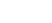whereis synchronous speed or the stator electrical speed in RPM,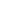is the power system frequency,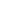is the number of magnetic poles in the machine.

Slip in the machine is calculated by: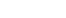whereis the slip,is synchronous speed or the stator electrical speed in RPM,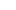is the rotor mechanical speed in RPM. Values of slip are tabulated and used for subsequent computations.

The impedance of the machine determined by solving the equivalent circuit for the Thévenin equivalent circuit. At the terminals of the machine the impedance is calculated by :An important consequence of this equation is the impedance of the machine is a function of the rotor slip. Knowing the slip and the impedance, the current in the stator can be calculated by: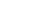where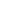is the stator current,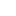is the terminal voltage: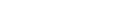andis the machine impedance.

In an induction machine operating below synchronous speed (as a motor) the real component of the stator currentand the imaginary component are supplied by the power system. If the induction machine is operating above synchronous speed (as a generator) the real component of the stator currentis exported back to the power system while the imaginary component is still supplied by the power system. The two scenarios are expressed where the sign of $I_{real}$ flips :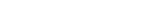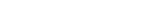After solving for the impedance of the machine over the range of rotor slips and then calculating the stator current at each slip, the real and reactive power consumed or exported by the machine is found. To calculate the real and reactive power: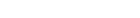where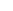is real power consumed or generated by the machine,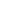is reactive power consumed or generated by the machine,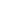is the line-to-line voltage, 13.8 kV,is the stator current and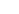is the power factor or the phase angle of. 

Values computed using are plotted in below. Figures induction_generator.pdf and induction_generator_zoom.pdf focus on the rotor angular velocity where the machine transitions from motor to generator.

At synchronous speed,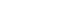RPM, the stator current, real and reactive power all approach to zero. At this speed the slip is zero and the machine is idling, neither consuming or exporting energy. Above synchronous speed,RPM, the real power reverses polarity while the reactive power retains the same polarity. The machine is now acting as a generator, exporting real power and importing reactive power. In all three figures positive power values are consumed by the machine while negative power values are exported from the machine.Plot of the real power, reactive power and stator current consumed or exported by the machine as calculated by Equations \ref{eq:induction_machine_real_power} and \ref{eq:induction_machine_reactive_power}. Positive power values are consumed by the machine while negative power values are exported from the machine. At 1800 RPM the transition from motor to generator is apparent.Enlarged plot of when the machine is operating as a generator. At synchronous speed, $n_s=1800$ RPM, the stator current approaches to zero. Above synchronous speed, $n_s>1800$ RPM, the real power reverses polarity while the reactive power retains the same polarity. Positive power values are consumed by the machine while negative power values are exported from the machine.Enlarged plot of the transition region between motor and generator operation. Positive power values are consumed by the machine while negative power values are exported from the machine.

## References

 S. J. Chapman, Electric Machinery Fundamentals, Fourth. 1221 Avenue of the Americas, New York, NY 10020: McGraw-Hill, 2005.

 J. E. Barkle and R. W. Ferguson, “Induction generator theory and application,” Transactions of the American Institute of Electrical Engineers. Part III: Power Apparatus and Systems, vol. 73, no. 1, Jan. 1954.

Note: This is a revised, WordPress friendly adaptation of content from Brendon Bruns’ Petroleum Engineering Masters project: https://scholarworks.alaska.edu/handle/11122/10947